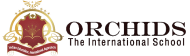Enquire Now

The result is:

Addition is a basic arithmetic operation used in our daily life. An addition calculator makes your task simpler and faster, giving accurate results in significantly less time. It is a free online tool which helps in doing large calculations. While calculating our monthly expenses or grocery bills, addition is one of our most frequent operations.

In mathematics, addition is the process of combining two or more values. It is the process of combining various values into one sum. The addition is denoted by the symbol "+".
In the addition procedure, the values are called addends and the resultant is known as the sum. Such as 2 + 1 = 3, where 2 and 1 are the addends, and 3 is the sum.
1. Find the value.
82 + 65 = ?
To perform addition in the basic calculator. Enter all the given values using the addition operator (+), i.e.,
82 + 65 = 147
2. Find the value.
67 + 85 + 99 + 76 + 119 + 34 = ?
To perform addition in the basic calculator. Enter all the given values using the addition operator (+), i.e.,
67 + 85 + 99 + 76 + 119 + 34 = 480
How to Use an Addition Calculator?